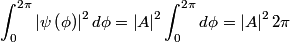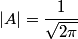## Solution to 1986 Problem 28\begin{align*}\int_0^{2\pi} \left| \psi\left(\phi \right) \right|^2 d \phi = \left|A\right|^2 \int_0^{2\pi} d \phi = \left|A\...Thus, in order for this to be equal to 1, we must have that\begin{align*}\left|A\right| = \frac{1}{\sqrt{2 \pi}}\end{align*}So, answer (D) is correct.